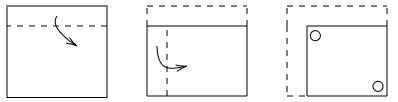Search
•APSEd

# GATE 2021 - New Syllabus | Additional Concepts for GATE CE

GATE 2021 syllabus is slightly modified compared to the previous years. Here, I am going to set five questions which are brand new and from the new syllabus. The concepts of these questions were not a part of previous years' GATE, so you need to be extra focused on these.

All of you have invested so much of time and energy for GATE Preparation. Is it not? But, do you know preparation without practice has no point.

Practising is the fundamental step of reducing negative marks and ultimately it makes you score your target. It also helps you understand how to skip a question, which question to be skipped, and how much time the question is going to take.You can take the quiz as a part of your last-minute preparation. Come on, turn your stopwatch on, just to keep a time track. Ready, Set, Let's Go!

### Question 1

If the 2 lines of regression are given as 3x - 2y + 1 = 0 and 2x - y - 2 = 0, find the means of x and y variables respectively

(A) 8 and 5

(B) 5 and 5

(C) 8 and 8

(D) 5 and 8

### Question 2

MSQ: In an undamped single degree of freedom system, the stiffness is 50 N/mm, the maximum amplitude observed is 5 cm and the frequency of the vibration is 10 Hz. Which of the following option is/are correct?

NOTE: This is a multiple select question, one or more than one option can be correct.

(A) Maximum velocity = 3.14 m/s

(B) Total energy of motion = 62.5 J

(C) Maximum acceleration = 300 m/s ^2

(D) Mass of body = 0.126 kg

### Question 3

NAT: If the 2 coefficients of regressions are -0.8 and -1.3, the coefficient of correlation is _____ (round up to 2 decimal places).

### Question 4

Which of the following options would be most appropriate regarding the unfolded shape form of the figure?(A) In the unfolded figure, there will be a total of 6 circular holes

(B) In the unfolded figure, there will be a total of 4 circular holes

(C) In the unfolded figure, four circular holes will be at the left-hand top corner

(D) In the unfolded figure, four circular holes will be at the left-hand bottom corner

### Question 5

What are the allowable 24 hours weighted SO2 concentration in 𝛍g/m^3 in industrial and ecologically sensitive areas respectively?

(A) 50 and 54

(B) 50 and 50

(C) 80 and 80

(D) 80 and 54

How much time did you take? I hope you finished it before 15 minutes!

## Hints

Did you face any difficulty? I will give here the hints to solve these questions, but not the whole explanation and answer.

### Question 1

This is a simple but tricky question on linear regression. The only concept you have to use here is "the intersection point of two regression lines is the means of their variables".

Simplifying to well enough, if the regression lines are the functions of X and Y, then the intersection point of these regression lines will be Xm, Ym.

Here Xm and Ym are the means of X and Y.

### Question 2

In an undamped single degree of freedom system,

• The total energy of motion = 1/2 x k x A^2

• Frequency of motion = 1/2π x sqrt(k/m)

• Maximum velocity = A x sqrt(k/m)

• Maximum acceleration = A x (k/m)

Where, A: Amplitude

k: Spring constant

m: mass of body

### Question 3

This is 'a use the formula and get the answer' type question. It should not have taken more than a minute.

Concept 1: Coefficient of correlation = sqrt(regression coefficient of x on y * regression coefficient of y on x

Concept 2: All these three coefficients carry the same sign

### Question 4

I will leave it to you. This is a mental ability question which comes under spatial aptitude section.

To win over these type of questions take a small piece of paper and fold/unfold it as the question says.

### Question 5

To answer this question you need to know the ambient air quality standard of India.

Before you leave, we have free weekly tests for GATE Civil like this in our app and web platform.

You can take it here.

### Be honest!

Keep your body, mind and emotions prepared for the exam day. We always are with you, in case of any queries feel free to contact us, the comment box is all yours!

Subscribe to APSEd Blog and get to know the latest.